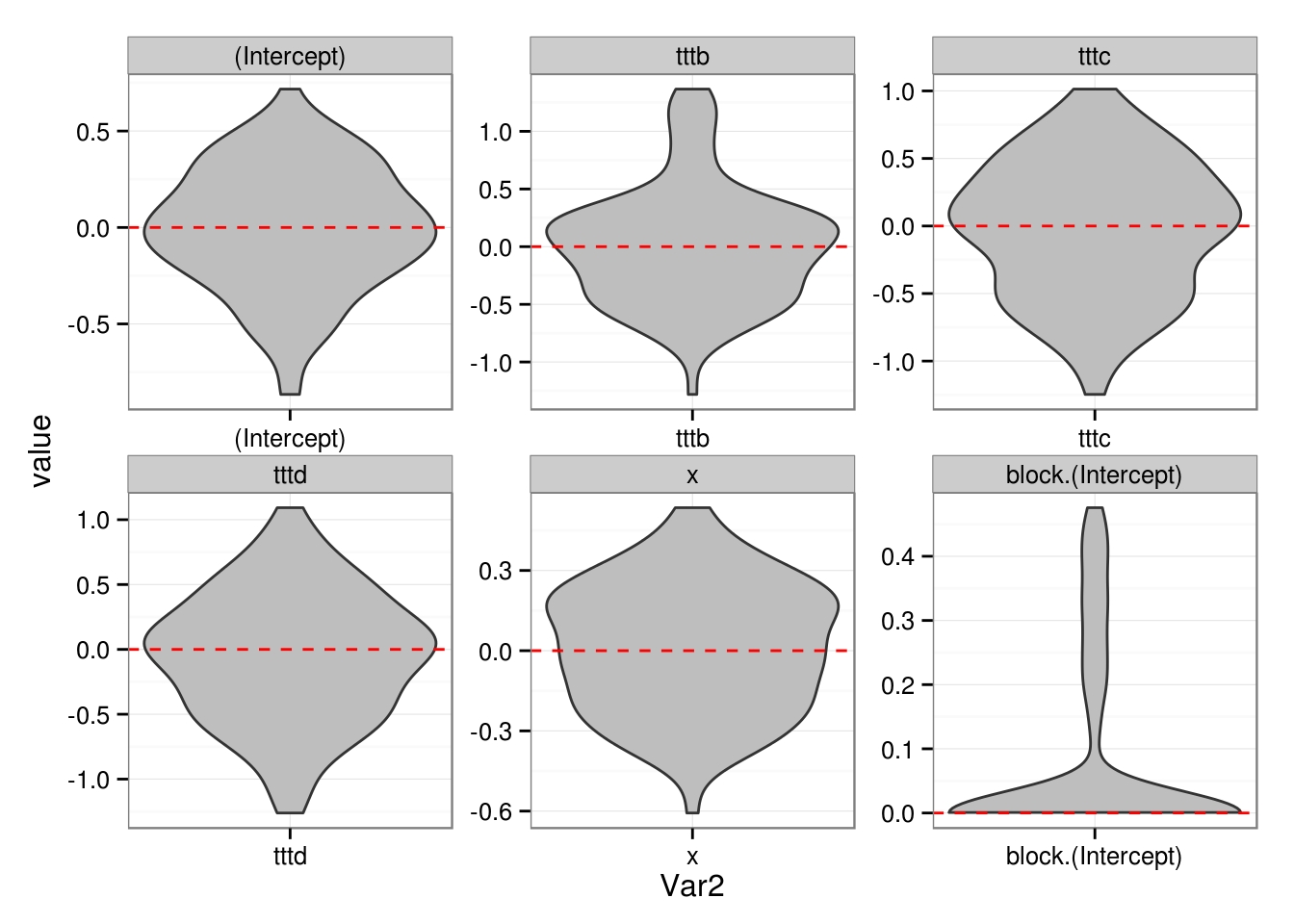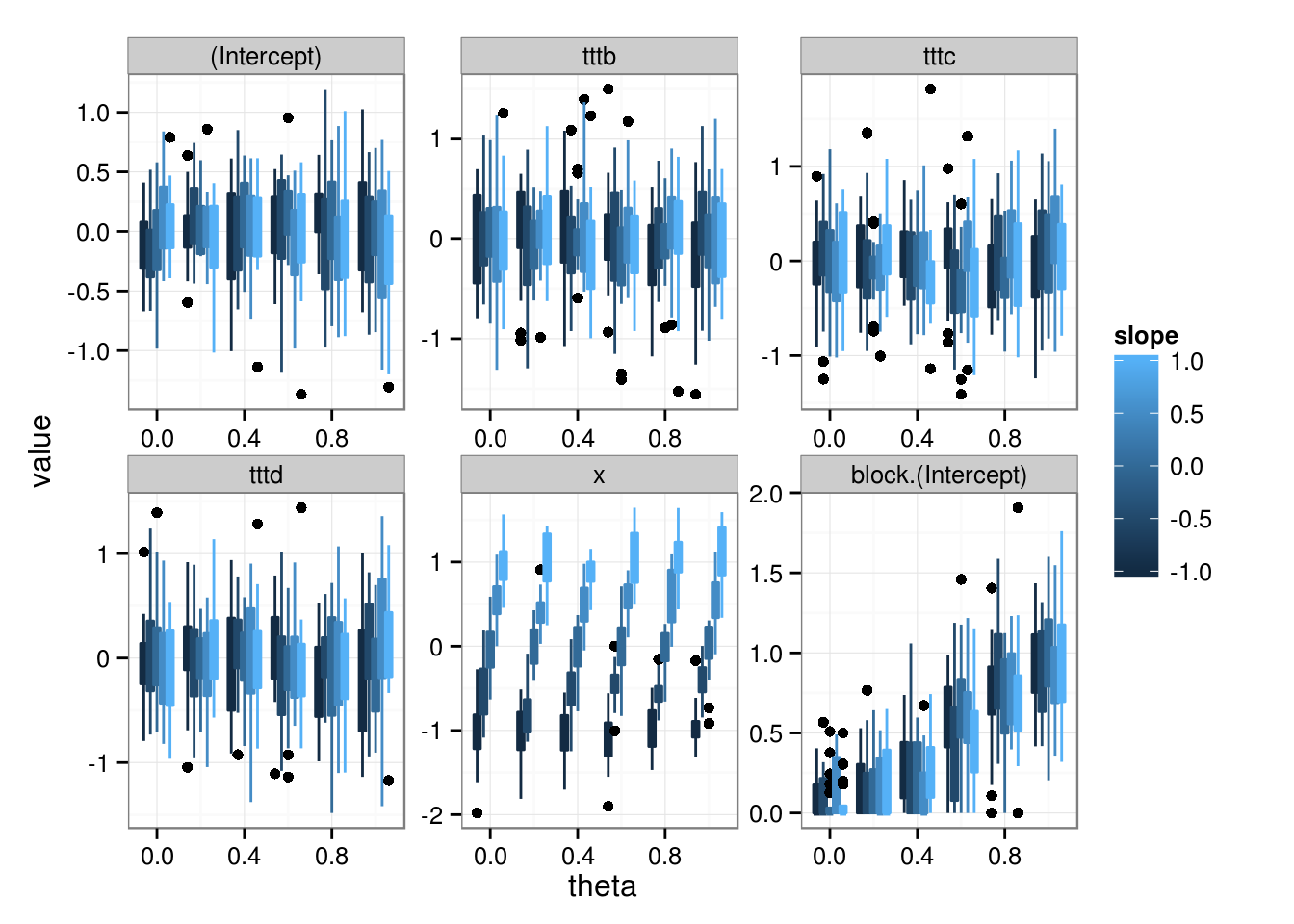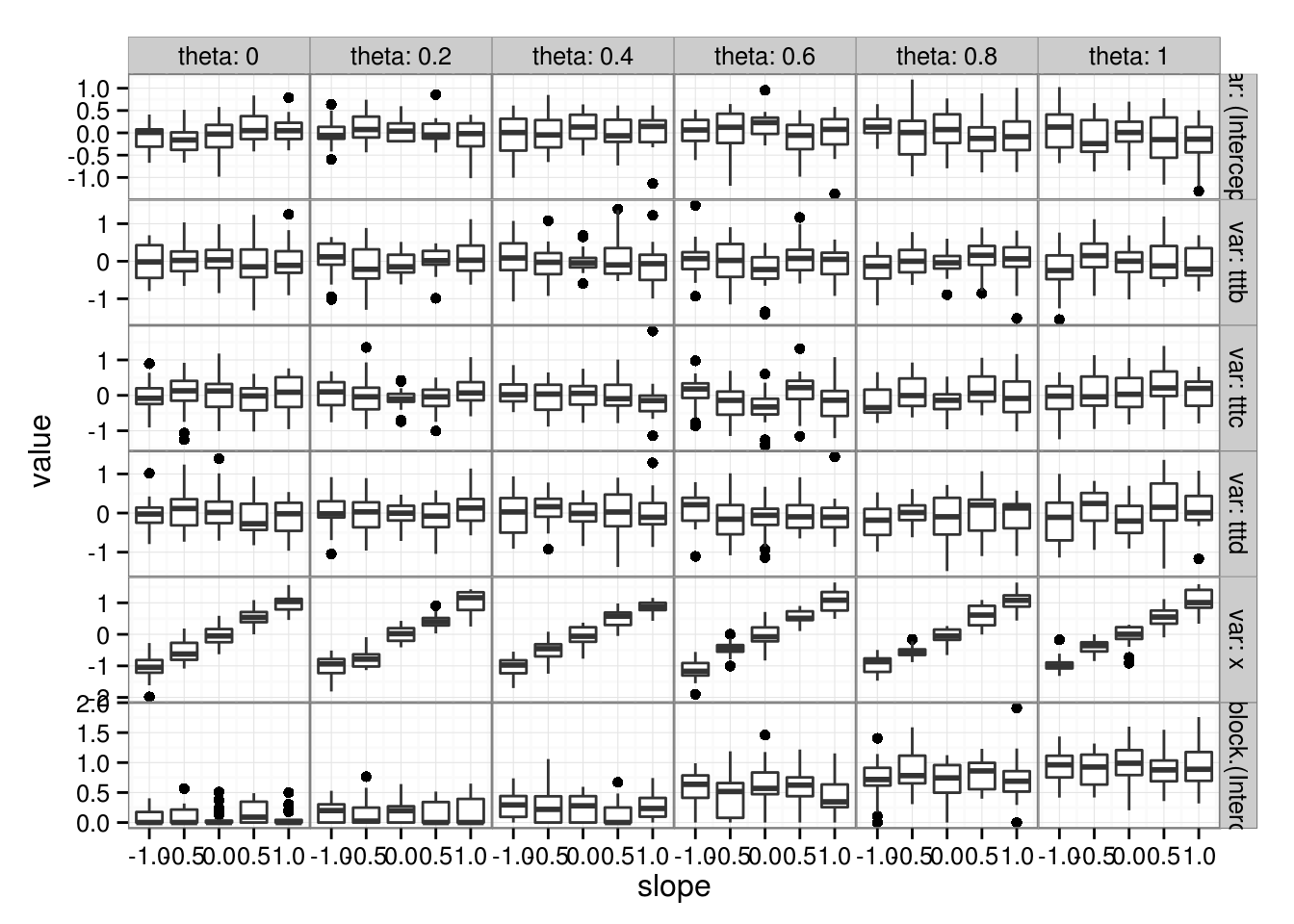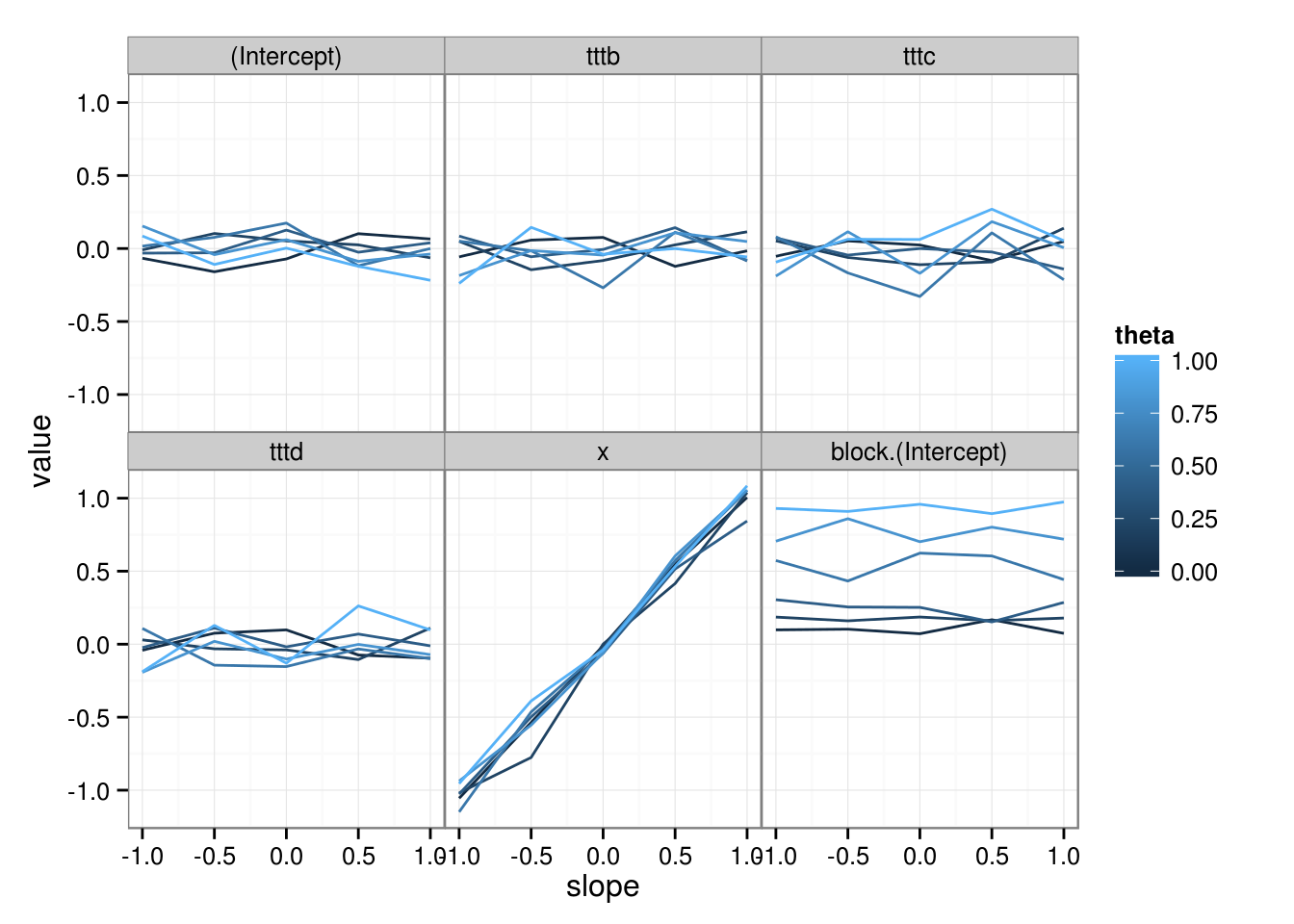# More lme4 simulation examples

Simulation is hard to pin down because there are so many possible axes of decision, but there are some tricks that make it easier. (Chapter 5 of Bolker 2008 has some examples, but they’re simultaneously less complicated [simpler experimental designs] and more complicated [nonlinear responses] than the examples here.)

library(reshape2) ## for melt()
library(plyr) ## for raply()
library(ggplot2); theme_set(theme_bw())
library(grid) ## for unit()
zmargin <- theme(panel.margin=unit(0,"lines"))
library(lme4)

## Experimental design/covariates

Here are some of the properties of the simulation that you have to decide on. It’s easier if you have a specific, real example in mind to guide you.

### Fixed effects

• how many fixed effects?
• categorical (factor) or continuous?
• continuous variables: evenly spaced (regression design), or evenly spaced with replication, or randomly distributed (what distribution, with what parameters)? Correlations among parameters?
• useful functions: expand.grid, runif, rnorm, seq, rep
• categorical variables: how many levels? Balanced sample, or random sample of levels? Crossed or nested design?
• useful functions: expand.grid, sample, rep, gl (maybe rpois for number of samples within levels)
• interactions: how are continuous covariates distributed within levels of categorical variables?
• for an unbalanced design, one trick is to construct a balanced design and then subsample

### Random effects

• how many random effects? how many levels of each?
• crossed, nested?
• interactions with fixed effects (in design: e.g. randomized block (replicated or unreplicated), split-plot, nested?)

### Response

• conditional distribution (Gaussian, binomial, Poisson, …)
• link function (typically choose canonical link for a given conditional distribution)
• for binomial sample: what is $$N$$?
• parameters: $$\beta$$, $$\theta$$, $$\sigma$$ (for LMMs)

### Example

Here I’ll do an example somewhat like the Culcita example. Here there are a specified number of random-effects groups with each treatment replicated multiple times within a block; in addition, there’s a continuous covariate (uniformly distributed) that has a unique value for every observation. The data are binomial with a user-specified $$N$$ (I don’t know what will happen if $$N$$ (N.binom in the function below) is specified as a vector: can simulate.formula handle a vector-valued size argument??)

For convenience in running multiple simulations I’ll wrap everything in functions whose parameters can easily be varied.

The simulation function:

simfun <- function(n.blocks=10,n.ttt=4,n.rep=4,N.binom=1,
x.range=c(-1,1),
## intercept, ttt effects, slope
## default gives homogeneous log-odds of 0 -> prob=0.5
beta=c(0,rep(0,n.ttt-1),0),
theta=0,
seed=NULL) {
if (!is.null(seed)) set.seed(seed)
dd <- expand.grid(block=factor(seq(n.blocks)),
ttt=factor(letters[seq(n.ttt)]),
rep=seq(n.rep))
dd$x <- runif(nrow(dd),min=x.range,max=x.range) dd$N <- N.binom
## the messages about "theta/beta parameter vector not named"
##  are annoying ...
dd$y <- suppressMessages(simulate(~ttt+x+(1|block),family="binomial", size=N.binom, newdata=dd, newparams=list(beta=beta,theta=theta))[]) return(dd) } A function for fitting the data. If it weren’t for x the experimental design would stay fixed and we could use refit, but we can’t (it is theoretically possible to adapt refit to this situation, but it would take a bit of hacking). fitfun <- function(dd) { glmer(y/N~ttt+x+(1|block), weights=N,family="binomial", data=dd) } And a function to generate summary output from a fit: sumfun <- function(fit) { c(fixef(fit),getME(fit,"theta")) } We can use raply from the plyr package simres <- raply(100,sumfun(fitfun(simfun()))) true_vals <- data.frame(Var2=c("(Intercept)", paste0("ttt",letters[2:4]), "x","block.(Intercept)"), value=c(0,0,0,0,0,0)) ggplot(melt(simres),aes(x=Var2,y=value))+geom_violin(fill="gray")+ facet_wrap(~Var2,scale="free")+ geom_hline(data=true_vals,aes(yintercept=value),col=2,lty=2)When I want to run a factorial simulation experiment (varying values for several different parameters), I usually prefer to run a series of nested for loops to fill in an array (although I may use raply, sometimes with .progress="text", for the inner loop that loops over replicates of the same parameter values). I typically set up the array first with carefully assigned dimnames so that it can be melted: my array dimensions are usually {par1,par2,...,parN,rep,vars}, where the last dimension is the vector of variables saved for each run. For example: thetavec <- seq(0,1,by=0.2) slopevec <- seq(-1,1,by=0.5) res0 <- sumfun(fitfun(simfun())) nrep <- 20 resarr <- array(dim=c(length(thetavec),length(slopevec),nrep,length(res0)), dimnames=list(theta=thetavec,slope=slopevec,rep=seq(nrep), var=names(res0))) beta.base <- rep(0,5) names(beta.base) <- names(res0)[1:5] ## prefer to use i,j,k,... for indices for (i in seq_along(thetavec)) { for (j in seq_along(slopevec)) { ## if you want to see progress in the output file ## cat(i,j,"\n") ## fancier progress bars available: ?txtProgressBar beta <- beta.base beta["x"] <- slopevec[j] resarr[i,j,,] <- raply(nrep, sumfun(fitfun(simfun(theta=thetavec[i], beta=beta)))) save("resarr",file="lme4_sims.RData") ## checkpointing ## decide whether you want this in the innermost or next- ## or next-next ... probably don't need to save more than once every ## 10 minutes or so unless you're really impatient and want ## to look at interim results in an independent R session } } There are various ways of saving long runs. * For the simplest, shortest kinds of runs, you can use knitr’s caching facility (just add cache=TRUE to the chunk options) * For longer runs, I often do my own version of caching by defining a file name (e.g. fn) and then using code structured as if (file.exists(fn)) load(fn) else { ...; save(fn)}. The simulations won’t be re-run if you change the code, but they will if you remove (or rename) the output file. * Alternatively, you may want to put the batch code in a completely separate .R file (you still need to save the .RData file from the batch run and use load() when you want to analyze the results). At this point you have a fairly complex object (a 4-dimensional,$6 5 20 6 array), but at least one that is fairly well labeled:

dimnames(resarr)
## $theta ##  "0" "0.2" "0.4" "0.6" "0.8" "1" ## ##$slope
##  "-1"   "-0.5" "0"    "0.5"  "1"
##
## $rep ##  "1" "2" "3" "4" "5" "6" "7" "8" "9" "10" "11" "12" "13" "14" ##  "15" "16" "17" "18" "19" "20" ## ##$var
##  "(Intercept)"       "tttb"              "tttc"
##  "tttd"              "x"                 "block.(Intercept)"

You can calculate summary statistics across various dimensions, especially across the replication dimension:

resmeans <- apply(resarr,c(1,2,4),mean,na.rm=TRUE)
resci <- apply(resarr,c(1,2,4),quantile,c(0.025,0.975))

Or draw pictures, after melt()ing the data (melting arrays with sensible dimnames automatically produces sensible results):

mres <- melt(resarr)
ggplot(mres,aes(theta,value,colour=slope))+
geom_boxplot(aes(fill=slope,
group=interaction(theta,slope)))+
facet_wrap(~var,scale="free")or

ggplot(mres,aes(slope,value))+
geom_boxplot(aes(group=slope))+
facet_grid(var~theta,scale="free",
labeller=label_both)+
zmarginor

ggplot(melt(resmeans),aes(slope,value,colour=theta,
group=theta))+
geom_line()+facet_wrap(~var)+zmarginOther handy tricks include

• superimposing the true values, e.g. using a data argument with a data frame composed of the true values;
• using violin plots (geom_violin) to summarise large sets of runs
• for runs with confidence intervals included in the summary, you can compute coverage (by sweep()ing the array with a comparison operator and the true values)
• for runs with $$p$$-values included in the summary, you can compute type I error and/or power by computing the mean(p<0.05) across the replication dimension
• you can use similar tricks to compute bias and RMSE when you have the true values (sweep() is handy again here)
• caterpillar plots (plots of replicates ordered by estimate value, including confidence intervals) are sometimes nice, although they can often be adequately summarized by power/coverage/etc.